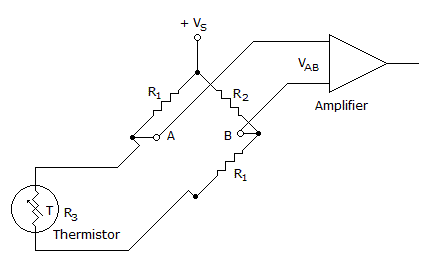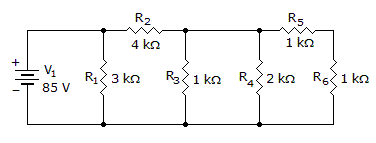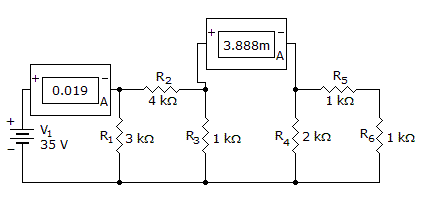# Electronics - Series-Parallel Circuits

### Exercise :: Series-Parallel Circuits - True or False

11.

If the output voltage of a certain loaded voltage divider is 12 V, the output voltage might decrease when the load is removed.

 A. True B. False

Explanation:

No answer description available for this question. Let us discuss.

12.If the Wheatstone bridge is balanced in the given circuit, the voltage between points A and B (VAB) equals the source voltage (VS).

 A. True B. False

Explanation:

No answer description available for this question. Let us discuss.

13.

The current through R6 is 8.5 mA.A. True B. False

Explanation:

No answer description available for this question. Let us discuss.

14.

Observed voltages not being shared identify components in parallel in a series–parallel circuit.

 A. True B. False

Explanation:

No answer description available for this question. Let us discuss.

15.

R2 is open.A. True B. False